## sábado, 18 de marzo de 2023

### The Half-Angle Formulas: A Powerful Tool for Trigonometry Students

Let me tell you about an exciting discovery I made while exploring the theoretical importance of half-angle formulas in trigonometry. These formulas, often overlooked and underappreciated in comparison to the more well-known laws of sines, cosines, and tangents, have a wealth of untapped potential waiting to be discovered.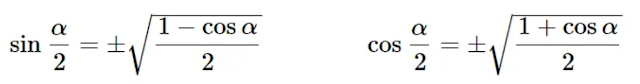The standard half-angle formulas

I'm not talking about the standard version of the half-angle formulas that appear in most introductory trigonometry textbooks. No, I'm referring to a version that relates the sides of a triangle, the perimeter, and its angles. These formulas have been largely ignored by the mathematical community, with even the almighty Wikipedia lacking an article on them.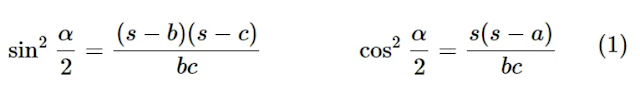The half-angle formulas for a triangle

My journey began when I stumbled upon (1) while attempting to prove the law of cosines by contradiction. I later discovered that this formula was already known, but that didn't stop me from exploring its potential. I went on to generalize the formula and discovered (2), which applies to cyclic quadrilaterals. When I found out that (2) was also already known, I refused to give up and continued to push the limits of these formulas.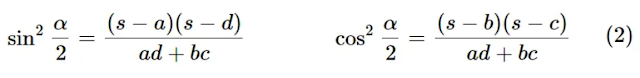The half-angle formulas for a cyclic quadrilateral

What I discovered next was truly mind-blowing. I managed to generalize (2) even further to (3), which applies to general quadrilaterals. Surprisingly, (3) also generalizes the Pythagorean trigonometric identity! These formulas not only provide a new framework for the Heron-Brahmagupta-Bretschneider development (see Two Identities and their Consequences, pp. 5), but they also have the potential to derive a plethora of other formulas and theorems, including the law of cosines, the law of sines, the law of tangents, and Stewart's theorem.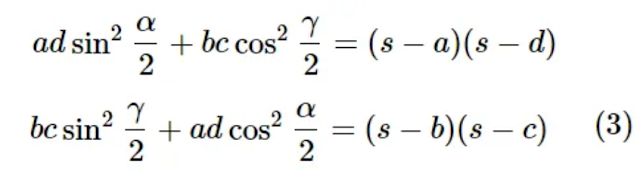The half-angle formulas for a general quadrilateral

And it doesn't stop there. Using these half-angle formulas, I also managed to derive a generalization of Mollweide's formula and a generalization of the law of tangents. The possibilities are endless, and I'm only scratching the surface of what these formulas can do.

But what excites me the most about these formulas is their potential to revolutionize the way we teach trigonometry to high school students. By introducing the half-angle formulas, we can help students better understand the development of the formulas that derive from them. This is where the half-angle formulas truly shine and can make a significant impact on math education.

In conclusion, the theoretical importance of the half-angle formulas cannot be overstated. They have the potential to unlock a whole new world of mathematical discoveries and have already proven to be a powerful tool for deriving a wide range of formulas and theorems. And with the right approach, they can also be a game-changer for teaching trigonometry to the next generation of students. The possibilities are truly endless, and I can't wait to see what else these formulas have in store for us.

## miércoles, 28 de diciembre de 2022

### Van Khea's areal problem

The following problem is a generalization of my previous problem conjectured by Van Khea. We will give a proof.

We will be using standard notation: $\lvert{BC}\rvert=a$, $\lvert{AC}\rvert=b$, $\lvert{AB}\rvert=c$; $\angle{BAC}=\alpha$, $\angle{ABC}=\beta$ and $\angle{BCA}=\gamma$. If $X$, $Y$ and $Z$ are the vertices of a triangle, we denote its area $[XYZ]$.

Problem. Let $ABC$ be a triangle and $P$ any point on the plane of $ABC$. Let $X$, $Y$ and $Z$ be arbitrary points on sides $BC$, $AC$ and $AB$, respectively. Let $D$ be the reflection of $P$ around $X$. Similarly, define $E$ and $F$. Denote $U$, $V$ and $W$ the midpoints of sides $BC$, $AC$ and $AB$, respectively. Let $D'$ be the reflection of $D$ around $U$. Similarly, define $E'$ and $F'$ (see figure below). Prove that

$$[DEF]+[D'E'F']=[ABC].$$

Proof. Denote $AZ=g$, $BZ=h$, $BX=j$, $CX=k$, $CY=l$ and $AY=m$. The area of ​​triangle $XYZ$ can be expressed as follows
$$[XYZ]=\frac12bc\sin{\alpha}-\frac12gm\sin{\alpha}-\frac12hj\sin{\beta}-\frac12kl\sin{\gamma}.$$
Since triangles $XYZ$ and $DEF$ are homothetic with scale factor $2$, it follows that $[DEF]=4[XYZ]$. Thus, we have
${[DEF]}=2bc\sin{\alpha}-2gm\sin{\alpha}-2hj\sin{\beta}-2kl\sin{\gamma}.\tag{1}$
Dividing both sides of $(1)$ by $[ABC]$ we have
$\frac{[DEF]}{[ABC]}=4\left[1-\left(\frac{gm}{bc}+\frac{hj}{ca}+\frac{kl}{ab}\right)\right].\tag{2}$
Segments $UX$ and $PD'$ are homothetic with center at $D$ and scale factor $2$. It follows that
$$PD'=2(j-\frac12a)=2j-a.$$
Similarly, we get $PE'=b-2l$ and $PF'=c-2g$. Since $UX$ and $PD'$ are homothetic segments, then $UX$ and $PD'$ are parallel and so are $VY$ and $PE'$. Hence $\angle{D'PE'}=\gamma$. Similarly, $\angle{D'PF'}=\beta$. So the area of triangle $D'E'F'$ is given by the expression
\begin{aligned}{[D'E'F']}&=[D'PE']+[D'PF']-[E'F'P]\\&=\frac{(2j-a)(b-2l)\sin{\gamma}}{2}+\frac{(2j-a)(c-2g)\sin{\beta}}{2}-\frac{(b-2l)(c-2g)\sin{(\beta+\gamma)}}{2}.\end{aligned}
Taking into account that $\sin{(\beta+\gamma)}=\sin{(\pi-\alpha)}=\sin{\alpha}$ and dividing by $[ABC]$ we obtain
$\frac{[D'E'F']}{[ABC]}=\frac{(2j-a)(b-2l)}{ab}+\frac{(2j-a)(c-2g)}{ca}-\frac{(b-2l)(c-2g)}{bc}.\tag{3}$
Adding equations $(2)$ and $(3)$, expanding and factorizing,
$$\frac{[DEF]}{[ABC]}+\frac{[D'E'F']}{[ABC]}=\frac{ca(4l+b)-4ga(m+l-b)-4j(b(g+h-c)+lc)-4klc}{abc}.$$
But $b=m+l$, $c=g+h$ and $a=j+k$, so
\begin{aligned}\frac{[DEF]}{[ABC]}+\frac{[D'E'F']}{[ABC]}&=\frac{ca(4l+b)-4jlc-4klc}{abc}\\&=\frac{ca(4l+b)-4cl(j+k)}{abc}\\&=\frac{ca(4l+b)-4cla}{abc}\\&=1.\end{aligned}
Therefore,
$$[DEF]+[D'E'F']=[ABC].$$
$\square$
Note: The point $P$ may cross the side lines of the triangle $ABC$ in points either interior or exterior to the sides. The reasoning in cases other than that considered above requires only minor adjustments.

## viernes, 23 de diciembre de 2022

### Areal property of the circumcircle mid-arc triangle

The following theorem is a property of the circumcircle mid-arc triangle which appears to be unknown.

We will be using standard notation: $\lvert{BC}\rvert=a$, $\lvert{AC}\rvert=b$, $\lvert{AB}\rvert=c$; $\angle{BAC}=\alpha$, $\angle{ABC}=\beta$ and $\angle{BCA}=\gamma$; $s$ for the semiperimeter; $R$ for the circumradius and $r$ for the inradius. If $X$, $Y$ and $Z$ are the vertices of a triangle, we denote its area $[XYZ]$.

Problem. Let $ABC$ be a triangle and $I$ its Incenter. Denote $\omega$ the circumcircle of $ABC$. Let $AI$ intersect $\omega$ again at $D$. Define $E$ and $F$ cyclically. Let $U$, $V$ and $W$ be the midpoints of $DE$, $EF$ and $FD$, respectively. Let $A'$ be the reflection of $A$ with respect to $V$. Define $B'$ and $C'$ cyclically (see figure 1). Then

$$[DEF]=[ABC]+[A'B'C'].\tag{1}$$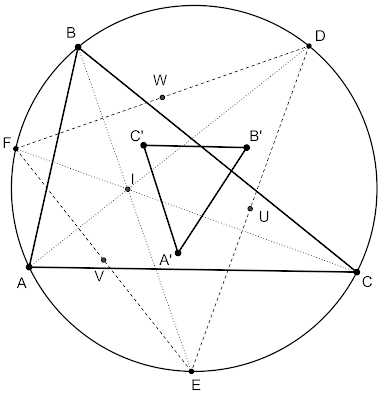Figure 1

Lemma 1. If $ABC$ is a triangle and $DEF$ is its circumcircle mid-arc triangle, then

$$\boxed{\frac{[ABC]}{[DEF]}=\frac{2r}{R}.}\tag{2}$$

Proof. By property of inscribed angles, $\angle{BAF}=\angle{ACF}=\frac12\gamma$ and $\angle{CAE}=\frac12\beta$. It follows that
\begin{aligned}&\angle{EAF}=\alpha+\frac12\beta+\frac12\gamma\\&=\pi-\beta-\gamma+\frac12\beta+\frac12\gamma\\&=\pi-\frac12(\beta+\gamma).\end{aligned}
By the law of sines,
$$\frac{EF}{\sin{(\pi-\frac12(\beta+\gamma))}}=\frac{EF}{\sin{\frac12(\beta+\gamma)}}=\frac{AF}{\sin{\frac12\gamma}}.\tag{3}$$
Since $\angle{AFC}=\beta$ and, again, by the law of sines,
$$\frac{AF}{\sin{\frac12\gamma}}=\frac{b}{\sin{\beta}}.\tag{4}$$
From $(3)$ and $(4)$ we get
$$EF=\frac{b\sin{\frac12(\beta+\gamma)}}{\sin{\beta}}=2R\sin{\frac12(\pi-\alpha)}=2R\cos{\frac12\alpha}.\tag{5}$$
Analogously, we can find that
$$DF=2R\cos{\frac12\beta}\qquad ED=2R\cos{\frac12\gamma}.\tag{6}$$
Note that $\angle{DFC}=\frac12\alpha$ and $\angle{EFC}=\frac12\beta$, so $\angle{DFE}=\frac12(\alpha+\beta)$. Then the area of $DEF$ is given by
$$[DEF]=\frac{EF\cdot{DF}\sin{\frac12(\alpha+\beta)}}{2}=2R^2\cos{\frac12\alpha}\cos{\frac12\beta}\cos{\frac12\gamma}.\tag{7}$$
Elsewhere we have proved that $s=4R\cos{\frac12\alpha}\cos{\frac12\beta}\cos{\frac12\gamma}$ (for a proof, see section 3, c), here). As the area of $ABC$ can also be written as $[ABC]=rs$, we can rewrite it like this
$$[ABC]=4Rr\cos{\frac12\alpha}\cos{\frac12\beta}\cos{\frac12\gamma}.\tag{8}$$
Dividing $(8)$ by $(7)$ we get
$$\frac{[ABC]}{[DEF]}=\frac{2r}{R}.$$
$\square$
Lemma 2. Let $ABC$ be an triangle and $H$ its orthocenter. Let $l_a$, $l_b$ and $l_c$ be the perpendicular bisectors of sides $BC$, $AC$ and $AB$, respectively. Denote $H_1$, $H_2$ and $H_3$ the reflections of $H$ around $l_a$, $l_b$ and $l_c$, respectively. Then
$$\boxed{\frac{[H_1H_2H_3]}{[ABC]}=\frac{(a^2-b^2)(a^2-c^2)}{b^2c^2}+\frac{(a^2-c^2)(b^2-c^2)}{a^2b^2}-\frac{(a^2-b^2)(b^2-c^2)}{a^2c^2}.}\tag{9}$$
Proof. From figure 2, note that
$$HH_3=2(\frac{c}{2}-AC_1).\tag{10}$$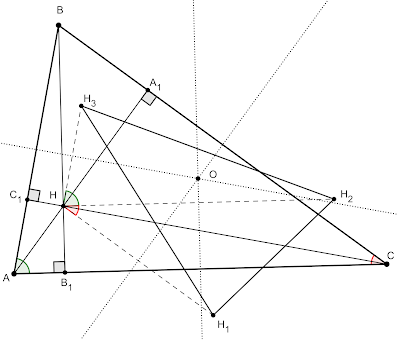Figure 2

Let $CH$ intersect $AB$ in $C_1$. Applying the law of sines in the triangle $ACC_1$, we have that
$$b=\frac{AC_1}{\sin{(\frac{\pi}{2}-\alpha)}}=\frac{AC_1}{\cos{\alpha}}.$$
So, $AC_1=b\cos{\alpha}$. Substituting in $(10)$ and then substituting from the law of cosines $\cos{\alpha}=\frac{b^2+c^2-a^2}{2bc}$ we have
$$HH_3=2(\frac{c}{2}-b\cos{\alpha})=c-2b\cos{\alpha}=\frac{a^2-b^2}{c}.$$
Similarly, we get that $HH_1=\frac{b^2-c^2}{a}$ and $HH_2=\frac{a^2-c^2}{b}$. Now, note that
$$[H_1H_2H_3]=[HH_2H_3]+[HH_1H_2]-[HH_1H_3].\tag{11}$$
As $HH_3$ and $HH_2$ are perpendicular to $l_c$ and $l_a$, respectively, then $\angle{H_2HH_3}=\alpha$ and similarly $\angle{H_2HH1}=\gamma$. Thus, equation $(11)$ can be written as follows
$$[H_1H_2H_3]=\frac{(a^2-b^2)(a^2-c^2)\sin{\alpha}}{2bc}+\frac{(a^2-c^2)(b^2-c^2)\sin{\gamma}}{2ab}-\frac{(a^2-b^2)(b^2-c^2)\sin{(\alpha+\gamma)}}{2ac}.\tag{12}$$
Dividing both sides of equation $(12)$ by $[ABC]=\frac12bc\sin{\alpha}=\frac12ab\sin{\gamma}=\frac12ac\sin{\beta}$ and taking into account that $\sin{(\alpha+\gamma)}=\sin{(\pi-\beta)}=\sin{\beta}$.
$$\frac{[H_1H_2H_3]}{[ABC]}=\frac{(a^2-b^2)(a^2-c^2)}{b^2c^2}+\frac{(a^2-c^2)(b^2-c^2)}{a^2b^2}-\frac{(a^2-b^2)(b^2-c^2)}{a^2c^2}.$$
$\square$
Note that the terms on the right hand side of the equation $(9)$ are the products of the Mollweide's formulas. Substituting from Mollweide's formulas and applying the identity of sine of double angle, we can write the equation $(9)$ as follows
$$\boxed{\frac{[H_1H_2H_3]}{[ABC]}=\frac{\sin{(\alpha-\beta)}\sin{(\alpha-\gamma)}}{\sin{\gamma}\sin{\beta}}+\frac{\sin{(\alpha-\gamma)}\sin{(\beta-\gamma)}}{\sin{\alpha}\sin{\beta}}-\frac{\sin{(\alpha-\beta)}\sin{(\beta-\gamma)}}{\sin{\alpha}\sin{\gamma}}.}\tag{13}$$

Back to the main problem
It is well-known that the orthocenter of the circumcircle mid-arc triangle is the incenter of the reference triangle. So $IB\perp{FD}$. Moreover, because of properties of inscribed angles, $$\angle{IDF}=\angle{FCB}=\angle{FDB}=\frac12\gamma$$
and $$\angle{IFD}=\angle{CAD}=\angle{DAB}=\angle{DFB}=\frac12\alpha.$$
Hence, by $ASA$, $DFI\cong{DFB}$. As a consequence, $I$ is the reflection of $B$ around $FD$. As $B'$ is the reflection of $B$ around $W$, it follows that the perpendicular bisector of $FD$ must also bisect $IB'$, meaning that $B'$ is the reflection of the orthocenter of $DEF$, $I$, around the perpendicular bisector of $DF$. Similarly, we conclude that $A'$ and $C'$ are the reflections of $I$ around the perpendicular bisectors of $EF$ and $DE$, respectively. Now our goal will be to show that $\frac{[A'B'C']}{[DEF]}=1-\frac{2r}{R}$. Note that $\angle{EFD}=\frac12(\alpha+\beta)$, $\angle{EDF}=\frac12(\beta+\gamma)$ and  $\angle{DEF}=\frac12(\alpha+\gamma)$. Applying formula $(13)$ to triangles $A'B'C'$ and $DEF$ and substituting angles we have
$$\frac{[A'B'C']}{[DEF]}=\frac{\sin{\frac12(\alpha-\gamma)}\sin{\frac12(\beta-\gamma)}}{\cos{\frac12\beta}\cos{\frac12\alpha}}+\frac{\sin{\frac12(\beta-\gamma)}\sin{\frac12(\beta-\alpha)}}{\cos{\frac12\gamma}\cos{\frac12\alpha}}-\frac{\sin{\frac12(\alpha-\gamma)}\sin{\frac12(\beta-\alpha)}}{\cos{\frac12\gamma}\cos{\frac12\beta}}. \tag{14}$$
Note that the right hand side of the equation $(14)$ are, again, products of the Mollweide's formulas. Thus, we have
\begin{aligned}\frac{[A'B'C']}{[DEF]}&=\frac{(a-c)(b-c)}{ab}+\frac{(b-c)(b-a)}{ac}-\frac{(a-c)(b-a)}{bc}\\&=1-\frac{c(a+b-c)}{ab}+1-\frac{b(a-b+c)}{ac}+1-\frac{a(-a+b+c)}{bc}.\end{aligned}\tag{15}
Substituting from the half-angle formulas
\begin{aligned}\frac{[A'B'C']}{[DEF]}&=3-\frac{2a\cos^2{\frac12\alpha}+2b\cos^2{\frac12\beta}+2c\cos^2{\frac12\gamma}}{s}\\&=1-\frac{a\cos{\alpha}+b\cos{\beta}+c\cos{\gamma}}{s}.\end{aligned}\tag{16}
Substituting from the law of sines, factorizing and applying double-angle identity for sine,
\begin{aligned}\frac{[A'B'C']}{[DEF]}&=1-\frac{R(\sin{2\alpha}+\sin{2\beta}+\sin{2\gamma})}{s}.\end{aligned}\tag{17}
But $\sin{2\alpha}+\sin{2\beta}+\sin{2\gamma}=4\sin{\alpha}\sin{\beta}\sin{\gamma}=\frac{abc}{2R^3}=\frac{2rs}{R^2}$, hence
$$\frac{[A'B'C']}{[DEF]}=1-\frac{R}{s}\left(\frac{2rs}{R^2}\right)=1-\frac{2r}{R}.\tag{18}$$
Finally, from $(2)$ and $(18)$,
$$[ABC]+[A'B'C']=\frac{2r}{R}[DEF]+\left(1-\frac{2r}{R}\right)[DEF]=[DEF].$$
$\square$
Some corollary inequalities:
• $\frac{(a^2-b^2)(a^2-c^2)}{b^2c^2}+\frac{(a^2-c^2)(b^2-c^2)}{a^2b^2}\geq\frac{(a^2-b^2)(b^2-c^2)}{a^2c^2}.$ (follows from $(9)$)
• $\frac{\sin{(\alpha-\beta)}\sin{(\alpha-\gamma)}}{\sin{\gamma}\sin{\beta}}+\frac{\sin{(\alpha-\gamma)}\sin{(\beta-\gamma)}}{\sin{\alpha}\sin{\beta}}\geq\frac{\sin{(\alpha-\beta)}\sin{(\beta-\gamma)}}{\sin{\alpha}\sin{\gamma}}.$ (follows from $(13)$)
• $\frac{c(a+b-c)}{ab}+\frac{b(a-b+c)}{ac}+\frac{a(-a+b+c)}{bc}\leq3.$ (follows from $(15)$)
• $a\cos{\alpha}+b\cos{\beta}+c\cos{\gamma}\leq{s}.$ (follows from $(16)$)
• $\sin{2\alpha}+\sin{2\beta}+\sin{2\gamma}\leq\frac{s}{R}.$ (follows from $(17)$)
• $R\geq{2r}.$ (This is Euler's Inequality! It follows from (18))
Relation $(1)$ seems like a good companion for property 4 of the Garcia-reflection triangle. See M. Dalcín, S. N. Kiss Some Properties of the García Reflection Triangles 119--126.

Update. Van Khea has generalized my problem. I have given a proof of his nice generalization which is available at Van Khea's areal problem.

## domingo, 7 de agosto de 2022

### A property of the Spieker point

Problem. Let $ABC$ be a triangle. Let $A'$ be on $BC$ such that $AA'$ is an internal angle bisector. Call $M$ the midpoint of $AA'$. Let $T$ be the point of tangency of $BC$ with the $A$-excircle. Prove that the Spieker center of $ABC$ lies on $MT$.

Proof. Let $D$, $E$ and $F$ be the midpoints of sides $AC$, $AB$ and $BC$, respectively. Let the angle bisector of $\angle{EDF}$ intersect $ET$ in $D'$ and $BC$ in $D''$. Similarly, let the angle bisector of $\angle{DEF}$ intersect $DT$ in $E'$, $AC$ in $E''$ and $BC$ in $E^*$. Call $S$ the intersection of $DD'$ and $EE'$. Since $AB\parallel{DF}$, a simple angle chase lead us to conclude that $\triangle{FDD''}$ is isosceles with $FD=FD''$. Moreover, since $DE\parallel{D''T}$ it follows that $\triangle{EDD'}\sim{\triangle{TD'D''}}$, from which we have

$\frac{ED'}{D'T}=\frac{DE}{D''T}=\frac{DE}{D''F+FT}=\frac{\frac{a}{2}}{\frac{c}{2}+\frac{a}{2}-(s-b)}=\frac{a}{b}.\tag{1}$
An analogous reasoning lead us to conclude that $\triangle{DEE''}$ is isosceles (with $DE=DE''$) and $\triangle{DEE'}\sim{\triangle{TE'E^*}}$, from which we obtain
$\frac{TE'}{E'D}=\frac{TE^*}{ED}=\frac{CT+CE^*}{ED}=\frac{CT+CE''}{ED}=\frac{(s-b)+\frac{b}{2}-\frac{a}{2}}{\frac{a}{2}}=\frac{c}{a}.\tag{2}$
Now, since $BC$ is the homothetic image of $ED$ with scale factor $2$, then $M$ must lies on $DE$ and by the Angle Bisector Theorem,
$\frac{DM}{ME}=\frac{AD}{AE}=\frac{b}{c}.\tag{3}$
We want to show that $EE'$, $DD'$ and $MT$ are concurrent, so by invoking the Ceva's Theorem and substituting from $(1, 2, 3)$,
$$\frac{ED'}{D'T}\cdot{\frac{TE'}{E'D}}\cdot{\frac{DM}{ME}}=\frac{a}{b}\cdot{\frac{c}{a}}\cdot{\frac{b}{c}}=1.$$
This means $EE'$, $DD'$ and $MT$ are concurrent at $S$. Hence $S$ lies on $MT$.
$\square$

## martes, 2 de agosto de 2022

### Solution of a problem by Tran Viet Hung

This is problem $037$ in this document by the Vietnamese geometer, Tran Viet Hung

Problem. Let $ABC$ be a triangle and $I$ its Incenter. Denote $D$, $E$ the points of tangency of incircle of $ABC$ with sides $BC$ and $AC$, respectively. Let $P$ be the intersection of the perpendicular of $AI$ at $A$ and $BC$. Construct $Q$ similarly. Denote $M$ and $N$ the midpoints of $AP$ and $BQ$, respectively. Prove that $MN$, $AB$ and $DE$ concur (see figure below).

Lemma 1. Let $P'$ be the intersection of $AI$ with $BC$ and $Q'$ the intersection of $BI$ with $AC$. Denote $M'$ and $N'$ the midpoints of $AP'$ and $BQ'$, respectively. Call $X$ the intersection of $AN'$ with $BM'$. If $T'$ is the point of tangency of the $C$-excircle with $AB$, then $T'$, $X$ and $I$ are collinear.

Proof. Here we'll be using standard notations, this is $BC=a$, $AC=b$, $AB=c$ and $s$ is semiperimeter. From the formula for the length of angle bisector we have
$$AP'=\frac{2\sqrt{bcs(s-a)}}{b+c}.\tag{1}$$
Moreover, it is well-known that
$$AI=\sqrt{\frac{bc(s-a)}{s}}.\tag{2}$$
From $(1)$ and $(2)$ follows that
$$M'I=AI-\frac{AP'}{2}=\sqrt{\frac{bc(s-a)}{s}}-\frac{\sqrt{bcs(s-a)}}{b+c}=\frac{1}{b+c}\cdot{\sqrt{\frac{bc(s-a)^3}{s}}}.$$
Similarly, we can derive an expression for $N'I$,
$$N'I=BI-\frac{BQ'}{2}=\sqrt{\frac{ac(s-b)}{s}}-\frac{\sqrt{acs(s-b)}}{a+c}=\frac{1}{a+c}\cdot{\sqrt{\frac{ac(s-b)^3}{s}}}.$$
Invoking Ceva's theorem, our goal now is to show that
$$\frac{AM'}{M'I}\cdot{\frac{N'I}{BN'}}\cdot{\frac{BT'}{AT'}}=1.\tag{3}$$
Since $AT'=(s-b)$ and $BT'=(s-a)$, equation $(3)$ can be re-written as
$$\frac{\sqrt{bcs(s-a)}}{\sqrt{\frac{bc(s-a)^3}{s}}}\cdot{\frac{\sqrt{\frac{ac(s-b)^3}{s}}}{\sqrt{acs(s-b)}}}\cdot{\frac{s-a}{s-b}}=\frac{s}{s-a}\cdot{\frac{s-b}{s}}\cdot{\frac{s-a}{s-b}}=1.$$
This means that $AN'$, $BM'$ and $IT'$ are concurrent at $X$. Hence $T'$, $X$ and $I$ must be collinear.
$\square$
Back to Hung's original problem
Call $I_c$ the $C$-excenter of $ABC$. By property of ex-centers, $\angle{IBI_c}=\angle{IAI_c}=90^\circ$, so $AP$ and $BQ$ must intersect at $I_c$. Denote $Y$ the intersection of $AN$ with $BM$ and $T$ the point of tangency of the incircle with $AB$. Then $Y$, $I_c$ and $T$ must be collinear since this is the extraverted version of Lemma 1. Now, suppose $DE$ and $MN$ intersect $AB$ at $R$ and $R'$, respectively. It is well-known that $AD$, $BE$ and $CT'$ are concurrent at the Gergonne Point, so by property of harmonic bundles we have
$$-1=(A, B; T, R)=(A, B; T, R'),$$
wich means $R=R'$, hence $MN$, $AB$ and $DE$ concur.
$\square$
Remark. Lemma 1 has given rise to a new special triangle, namely the Garcia-Moses triangle, published in the Encyclopedia of Triangle Centers.

## jueves, 21 de julio de 2022

### The jealous engineer problem

One day Tony woke up and didn't find his wife in the house.

— Where were you?

— I wanted to work out and just walked around the block. Look at my pedometer.

— What distance does the pedometer show?

—  Really Tony? again with your jealousy?

Angry, Tony snatches the pedometer from her and he read a distance traveled of $805$ meters.

— How many laps did you do?

— One lap!

— East or North?

— Are you serious, Tony?

— East or North?

— East!

Tony had been the engineer in charge of paving the block years ago, and he knew that the four streets that made up the block were the same distance from the church where he married his wife. In addition, he remembered that the corners formed the following sequence of angles until he got back to his house: $60°$, $135°$, $85°$, $80°$ and that if his wife went east she must have traveled $200$ meters on the first street before to reach the $60°$- corner, a distance that he also remembered perfectly.

### El problema del ingeniero celoso

Un día, Tony se despertó y no encontró a su esposa en la casa.

— ¿Dónde estabas?

— Quería ejercitarme y solo recorrí la cuadra. Mira mi podómetro.

— ¿Qué distancia marca el podómetro?

— ¿En serio, Tony? ¿otra vez con tus celos?

Airado, Tony le arrebata el podómetro y lee una distancia recorrida de $805$ metros.

— ¿Cuántas vueltas diste?

— ¡Una! ¡una vuelta!

— ¿Este o norte?

— ¿Hablas en serio, Tony?

— ¿Este o norte?

— ¡Este!

Tony había sido el ingeniero a cargo del asfaltado de la cuadra años atrás y sabía que las cuatro calles que formaban la cuadra estaban a la misma distancia de la iglesia donde se casó con su esposa. Además, recordaba que las esquinas formaban la siguiente secuencia de ángulos hasta llegar de vuelta a su casa: $60°$, $135°$, $85°$, $80°$ y que si su esposa partió hacia el este debió haber recorrido $200$ metros en la primera calle antes de llegar a la esquina de $60°$, distancia que también recordaba a la perfección.

¿Mentía la esposa? Justifica tu respuesta.